# Calculate the area and perimeter of the following shapes:Q.1 Calculate the area of Square and rectangle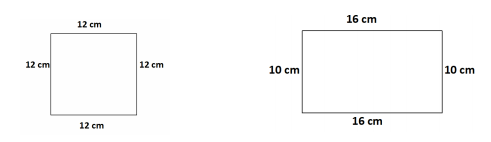Q.2 Calculate perimeter of Square and rectangle.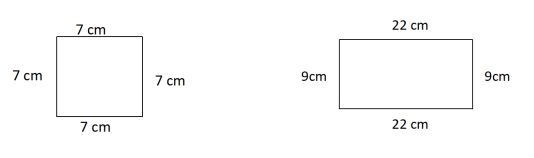"

1. Given: A Square and a rectangle.

To find: We have to find the area of the square and rectangle.

Solution: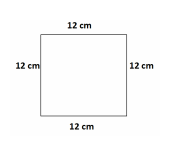Area of square = (Side length)2 = (12)2 cm2 = 144 cm2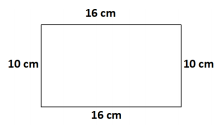Area of rectangle = Length x Breadth = 16 x 10 cm2 = 160 cm2

2. Given: A Square and a rectangle.

To find: We have to find the perimeter of the square and rectangle

Solution: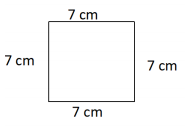Perimeter of square = 4 x (Side length) = 4 x 7 cm = 28 cm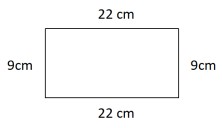Perimeter of rectangle = 2 x (Length + Breadth) = 2 x (22 + 9) cm = 2 x 31 cm = 62 cm